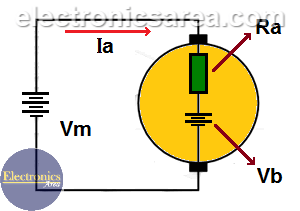DC Motor Counter-electromotive force – Load effect

Counter-electromotive force or Back-electromotive force

When a DC motor is powered, the supply voltage (Vm) is divided into:

• Voltage in the resistance of the DC motor windings (due to the internal resistance Ra) and …
• A voltage called: Couter-electromotive force (CEMF).

See the diagram, where:

• Vm = motor input voltage (volts)
• Ra = resistance of the excitation winding (ohms)
• Ia = excitation current (amps)
• Vb = Counter-electromotive force (CEMF) due to motor rotation (volts)Applying the Kirchhoff voltage law: Vm = Vb + (Ia x Ra) or Vb = Vm – (Ia x Ra)

From the last equation it is seen that when the value of current “Ia” increases, the value of “Vb” decreases.

Counter-electromotive force constant (K)

The counter electromotive force (CEMF) is proportional to the motor speed and the intensity of the magnetic field. If the DC motor has a permanent magnet rotor, this constant is: K = Vb / Nd, Where:

• K = counter-electromotive force constant (CEMF) of the motor, (Volts/rpm)
• Nd = motor speed (rpm)

Note: rpm = revolutions per minute.

Load effect on a DC motor

In a DC motor, the speed and current needed by the motor depend on the load. In this type of motor part of the applied voltage (Vm) is in the internal resistance (resistance of the excitation winding). The rest of the voltage (Vb) is used to spin the motor.

When the load increases, the current (Ia) increases. This current causes a higher voltage drop in the internal resistance of the motor (resistance of the excitation winding).

Since the voltage that powers the DC motor is constant, the voltage for turning the motor is lower and consequently the speed of the motor rotation is lower too. See the following formula: Vb = Vm – Ia x Ra, where:

• Vb: actual voltage used to spin the motor.
• Vm: voltage applied to the entire motor assembly.
• Ra: resistance of the excitation winding (internal resistance).
• Ia: current flowing through the DC motor.

Ia x Ra: is the voltage in the internal resistance of the DC motor. See that it depends directly on “Ia” (power supply current). If current “Ia” increases, Vb decreases and, as the speed of rotation of the motor is proportional to Vb, If Vb decreases then the motor speed too.

• 1
•
• 1
•
•
•
•
•
•
2
Shares
•
2
Shares
• 1
•
• 1
•
•
•
•
•